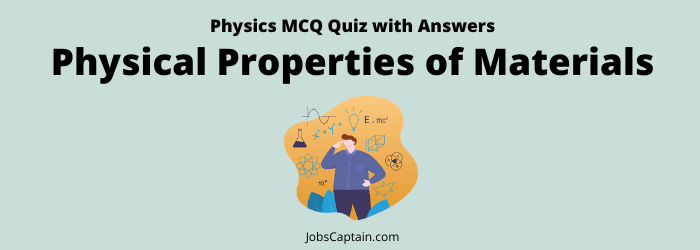# Physics: Physical Properties of Materials Quiz 2Quiz Set 1 2

Question 1. At which temperature density of water is maximum?

(A) 8ºC

(B) 4ºC

(C) 0ºC

(D) 4ºC

(D) 4ºC

Question 2. The maximum density of water is at _________.

(A) 269 Kelvin

(B) 273 Kelvin

(C) 277 Kelvin

(D) 373 Kelvin

(C) 277 Kelvin

Question 3. Why does an iron nail float on mercury while it sinks in water?

(A) Mercury is heavier than water

(B) Iron has greater density than water and lesser than mercury

(C) Iron is heavier than water and lighter than mercury

(D) Due to less chemical interaction of iron with mercury than it is with water

(B) Iron has greater density than water and lesser than mercury

Question 4. A needle sinks in water whereas a ship made of iron floats on it because?

(A) Specific gravity of the needle is greater than that of water displaced by it

(B) The ship driver, by power flat engine

(C) The ship is flat

(D) The edge of the needle is pointed

(A) Specific gravity of the needle is greater than that of water displaced by it

Question 5. An iron needle floats on the surface of water due to ________.

(A) Gravitational force

(B) Viscosity

(C) Surface tension

(D) Upthrust of water

(C) Surface tension

Question 6. While lifting a bucket of water from a well, we feel that the bucket?

(A) Has gained mass on coming out of water

(B)  Loses stability on coming out of the water

(C) Becomes lighter above the surface of water

(D) Becomes heavier above the surface of water

(D) Becomes heavier above the surface of water

Question 7. 200 gm of water is filled in a weightless balloon. Its weight in water will be?

(A) 400 gm

(B) 200 gm

(C) 100 gm

(D) Zero

(D) Zero

Question 8. The separation of cream from milk by churning is due to _________.

(A) Gravitational force

(B) Centrifugal force

(C) Cohesive force

(D)None of the above

(B) Centrifugal force

Question 9. The clouds float in the atmosphere because of their low?

(A) Density

(B) Pressure

(C) Velocity

(D) Temperature

(A) Density

Question 10. Which of the following elements was first produced artificially?

(A) Technetium

(B) Francium

(C) Plutonium

(D) Neptunium

(A) Technetium

Question 11. Static science is associated with __________.

(A) Data analyzing

(B) Mental situation

(C) Situation of rest

(D) Dynamic situation

(C) Situation of rest

Question 12. Consider the following statement. If there were no phenomenon of capillarity.

1. It would be difficult to use a kerosene lamp then.
2. One would not be able to use a straw to consume a soft drink.
3. The blotting paper would fail to function.
4. The big trees that we see around would not have grown on the Earth.

Which of the following statements given above are correct?

(A) Only 1, 2, 3 and 4

(B) Only 2 and 4

(C) Only 1, 3 and 4

(D) Only 1, 2 and 3

(C) Only 1, 3 and 4

Question 13. Mobiles and Automobiles have brought about a revolution in the social life of Indians, especially in the rural, in terms of

1. Mobility of the people
2. Connectivity of the people
3. Sensitivity of the people

Select the correct answer from the code given below.

(A) 1, 2 and 3

(B) Only 2 and 3

(C) Only 1 and 3

(D) Only 1 and 2

(D) Only 1 and 2

Question 14.

• Assertion (A): When a ship enters a sea from a river, it rises a little.
• Reason (R): The density of seawater is greater than that of river water.

In the context of the above statements, which of the following is true?

(A) (A) is false, but (R) is true

(B) (A) is true, but (R) is false

(C) Both (A) and (R) are true, but (R) is not the correct explanation of (A)

(D) Both (A) and (R) are true and (R) is the correct explanation of (A)

(D) Both (A) and (R) are true and (R) is the correct explanation of (A)

Question 15.

• Assertion (A): An iron ball floats on mercury but gets immersed in water.
• Reason (R): The specific gravity of iron is more than that of mercury.

(A) (A) is false, but (R) is true

(B) (A) is true, but (R) is false

(C) Both (A) and (R) are true, but (R) is not the correct explanation of (A)

(D) Both (A) and (R) are true and (R) is the correct explanation of (A)

(B) (A) is true, but (R) is false

Question 16. When a ship enters a sea from a river, what happens?

(A) It immersed in the bottom of the sea

(B) It sinks a little

(C) It remains at the same level

(D) It rises a little

(D) It rises a little

Question 17. Why it is easier to swim in the sea than in a river?

(A) The sea water is lighter

(B) The sea water is heavier

(C) The sea water is deep

(D) The sea water is salty

(D) The sea water is salty

Question 18. A body floats at 4ºC water. If temperature reaches 100ºC, then ________.

(A) The body will float freely

(B) More part of the body will submerge

(C) No change

(D) The body will sink

(D) The body will sink

Question 19. An ice cube is floating in a glass of water. What happens to the water level when the ice melts?

(A) First rises and then falls

(B) Remains the same

(C) Falls

(D) Rises

(B) Remains the same

Question 20. While floating in a sea what part of an iceberg is above the surface of the sea?

(A) 1/4

(B) 1/6

(C) 1/10

(D) 1/9